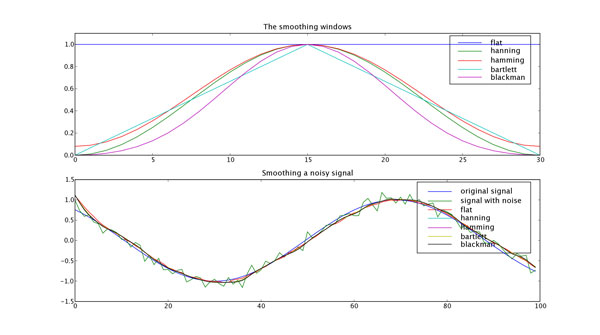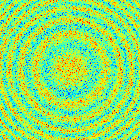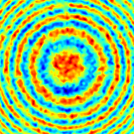# Smoothing of a 1D signal¶

This method is based on the convolution of a scaled window with the signal. The signal is prepared by introducing reflected window-length copies of the signal at both ends so that boundary effect are minimized in the beginning and end part of the output signal.

## Code¶

In [ ]:
import numpy

def smooth(x,window_len=11,window='hanning'):
"""smooth the data using a window with requested size.

This method is based on the convolution of a scaled window with the signal.
The signal is prepared by introducing reflected copies of the signal
(with the window size) in both ends so that transient parts are minimized
in the begining and end part of the output signal.

input:
x: the input signal
window_len: the dimension of the smoothing window; should be an odd integer
window: the type of window from 'flat', 'hanning', 'hamming', 'bartlett', 'blackman'
flat window will produce a moving average smoothing.

output:
the smoothed signal

example:

t=linspace(-2,2,0.1)
x=sin(t)+randn(len(t))*0.1
y=smooth(x)

numpy.hanning, numpy.hamming, numpy.bartlett, numpy.blackman, numpy.convolve
scipy.signal.lfilter

TODO: the window parameter could be the window itself if an array instead of a string
NOTE: length(output) != length(input), to correct this: return y[(window_len/2-1):-(window_len/2)] instead of just y.
"""

if x.ndim != 1:
raise ValueError, "smooth only accepts 1 dimension arrays."

if x.size < window_len:
raise ValueError, "Input vector needs to be bigger than window size."

if window_len<3:
return x

if not window in ['flat', 'hanning', 'hamming', 'bartlett', 'blackman']:
raise ValueError, "Window is on of 'flat', 'hanning', 'hamming', 'bartlett', 'blackman'"

s=numpy.r_[x[window_len-1:0:-1],x,x[-2:-window_len-1:-1]]
#print(len(s))
if window == 'flat': #moving average
w=numpy.ones(window_len,'d')
else:
w=eval('numpy.'+window+'(window_len)')

y=numpy.convolve(w/w.sum(),s,mode='valid')
return y

from numpy import *
from pylab import *

def smooth_demo():

t=linspace(-4,4,100)
x=sin(t)
xn=x+randn(len(t))*0.1
y=smooth(x)

ws=31

subplot(211)
plot(ones(ws))

windows=['flat', 'hanning', 'hamming', 'bartlett', 'blackman']

hold(True)
for w in windows[1:]:
eval('plot('+w+'(ws) )')

axis([0,30,0,1.1])

legend(windows)
title("The smoothing windows")
subplot(212)
plot(x)
plot(xn)
for w in windows:
plot(smooth(xn,10,w))
l=['original signal', 'signal with noise']
l.extend(windows)

legend(l)
title("Smoothing a noisy signal")
show()

if __name__=='__main__':
smooth_demo()


## Figure¶# Smoothing of a 2D signal¶

Convolving a noisy image with a gaussian kernel (or any bell-shaped curve) blurs the noise out and leaves the low-frequency details of the image standing out.

## Functions used¶

In [ ]:
def gauss_kern(size, sizey=None):
""" Returns a normalized 2D gauss kernel array for convolutions """
size = int(size)
if not sizey:
sizey = size
else:
sizey = int(sizey)
x, y = mgrid[-size:size+1, -sizey:sizey+1]
g = exp(-(x**2/float(size)+y**2/float(sizey)))
return g / g.sum()

def blur_image(im, n, ny=None) :
""" blurs the image by convolving with a gaussian kernel of typical
size n. The optional keyword argument ny allows for a different
size in the y direction.
"""
g = gauss_kern(n, sizey=ny)
improc = signal.convolve(im,g, mode='valid')
return(improc)


## Example¶

In [ ]:
from scipy import *

X, Y = mgrid[-70:70, -70:70]
Z = cos((X**2+Y**2)/200.)+ random.normal(size=X.shape)In [ ]:
blur_image(Z, 3)The attachment cookb_signalsmooth.py contains a version of this script with some stylistic cleanup.